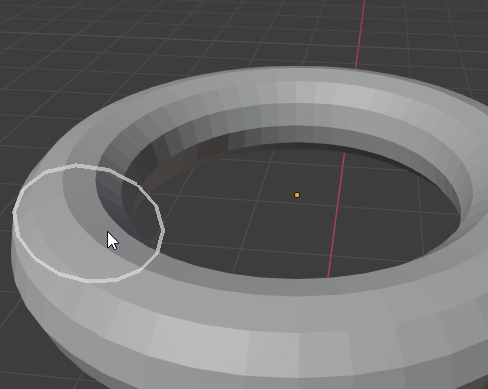# How to rotate a set of Vectors?

Hi, I am trying to rotate a list of vectors and was wondering how I can go about doing this.

So I create a circle like this:

``````(a, b, z) = (0,0,0) #position of circle

vertices = []

for angle in range(0, 360, 60):
print(angle)
vertices.append( (x,y,z))
``````

Is there a way I can translate the circle all while rotating the circles direction towards that same translation vector?

I am trying to achieve this old method which uses a real circle with vertices(worked fine but a bit of performance lag) so I’d like to switch to GPU shader. The vertices are assigned as the Draw shader “pos”If I’m understanding your question, what you want is to simulate rotating the circle around the 3D cursor. If that’s it, write code to:

• moves the cursor to the point around which you want to do the rotation,
• changes the Transform Pivot Point from Individual Origins to 3D Cursor, and
• does the rotation.

If I’ve misunderstood what you’re trying to do, please disregard this reply.I’m trying to align circle points to a vector( volume raycast ) while moving the circle location to match that same raycast location.

1 Like

You’re trying to simulate the pool of light cast while shining a flashlight around a room?